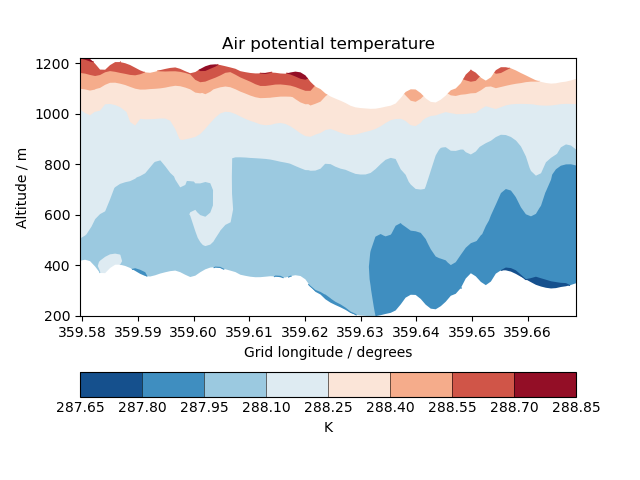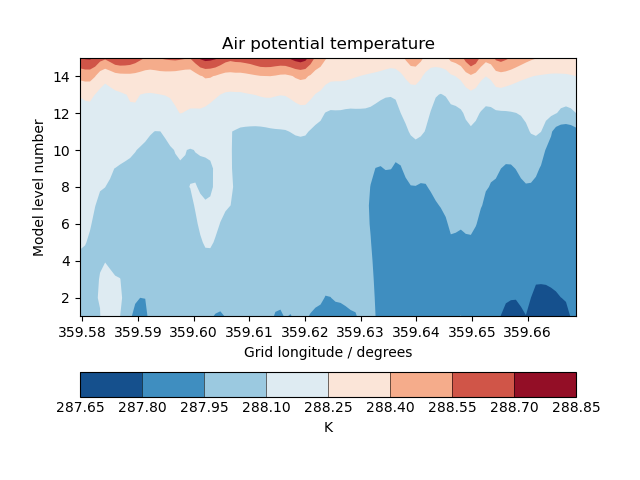# Cross Section Plots#

This example demonstrates contour plots of a cross-sectioned multi-dimensional cube which features a hybrid height vertical coordinate system.

••```import matplotlib.pyplot as plt

import iris
import iris.plot as iplt
import iris.quickplot as qplt

def main():
fname = iris.sample_data_path("hybrid_height.nc")

# Extract a single height vs longitude cross-section. N.B. This could
# easily be changed to extract a specific slice, or even to loop over *all*
# cross section slices.
cross_section = next(
theta.slices(["grid_longitude", "model_level_number"])
)

qplt.contourf(
cross_section, coords=["grid_longitude", "altitude"], cmap="RdBu_r"
)
iplt.show()

# Now do the equivalent plot, only against model level
plt.figure()

qplt.contourf(
cross_section,
coords=["grid_longitude", "model_level_number"],
cmap="RdBu_r",
)
iplt.show()

if __name__ == "__main__":
main()
```

Total running time of the script: (0 minutes 0.413 seconds)

Gallery generated by Sphinx-Gallery